# Module/rl obstacle avoidance

## Introduction

This module is a proof-of-concept for a new method for obstacle detection and avoidance on quadrotors. One that does not require the addition of any sensors, but relies solely on measurements from the accelerometer and rotor controllers. The detection of obstacles is based on the principle that the airflow around a quadrotor changes when the quadrotor is flying near a surface. A well-known example of this is the ground effect, an increase in lift force close to a ground surface. Similarly, a change in dynamics occurs when a quadrotor is flying close to a wall or ceiling. The proposed method uses a reinforcement learning controller to detect obstacles based on these measurements, and take action to lead the quadrotor back to safety.

Note: This is an initial proof-of-concept, limited to the detection of obstacles underneath a quadrotor.

The module has been demonstrated on the Parrot Bebop 1 and Parrot Bebop 2 quadrotors to be able to successfully detect and avoid obstacles underneath. It is expected that the method can be extended to the detection of obstacles above a quadrotor as well. Extension to surfaces on the same level as the quadrotor, e.g. walls, is expected to require some significant improvements to the algorithm.

The work that this module is based on can be found here: http://resolver.tudelft.nl/uuid:1ce67e4b-1e63-4e9f-8644-360642a11be6

## How does the algorithm work?

The detection of obstacles is based on a simplified quadrotor model and the estimation of an external force. In the current proof-of-concept, only the external force in the body z-axis is used for the detection of obstacles, but similar estimators can be derived for the external force in the body x and y-axis. This estimated external force in the body z-axis is used for the detection of obstacles underneath.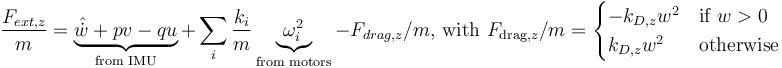$\frac{F_{ext,z}}{m}= \underbrace{\hat{\dot{w}} + pv - qu}_{\text{from IMU}} + \sum_i \frac{k_i}{m} \underbrace{\omega_i^2}_{\text{from motors}} - F_{drag,z}/m \text{, with } F_{\text{drag,}z}/m= \begin{cases} -k_{D,z} w^2 & \text{if } w > 0 \\ k_{D,z} w^2 & \text{otherwise} \end{cases}$

With:

•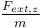${\textstyle \frac{F_{ext,z}}{m}}$, the estimated external force in z-direction (mass independent)
•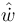${\textstyle \hat{\dot{w}}}$, the acceleration in the body z-axis, as provided by the onboard accelerometer
•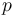${\textstyle p}$, the body roll rate
•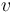${\textstyle v}$, the speed in body y-axis
•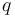${\textstyle q}$, the body pitch rate
•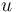${\textstyle u}$, the speed in body x-axis
•${\textstyle \frac{k_i}{m}}$, the propellor gains, estimated at the start of every flight
•${\textstyle \omega_i}$, the rotor speed in rad/s
•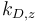${\textstyle k_{D,z}}$, the drag coefficient iin body z-axis, estiamted during a system identification experiment
•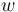${\textstyle w}$, the speed in body z-axis

## Reinforcement Learning

As the method is based on reinforcement learning, there are several hyperparameters that can be set. These can be set in the file: PAPARAZZI_HOME/sw/tools/rl_obstacle_avoidance/rl_obstacle_avoidance_critic.py. The following parameters are recommended as a starting point: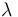${\textstyle \lambda}$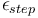${\textstyle \epsilon_{step}}$${\textstyle \alpha_0}$${\textstyle \epsilon_{episode}}$
Initial training 0.1 0.01 0.5 0.5 -> 0.0
Continuing training 0.1 0.01 0.1 0.01 -> 0.0

## Setup

### Airframe file

Add the following line to the airframe file to include the module.

<module name="rl_obstacle_avoidance"/>


To improve the performance it is recommended that the drag force is accounted for in the estimation of the external forces. In the module the drag force is assumed to be of the form: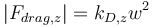$|F_{drag,z}| = k_{D,z} w^2$

The drag coefficient for the quadrotor being used be specified in the airframe file:

<section name="RL_OBSTACLE_AVOID" prefix="RL_OBSTACLE_AVOID_">
<define name="k_D_z" value="0.27106224"/>
</section>


The drag coefficients in body x-, y-, and z-axis can then be estimated in a system identification experiment. Some known coefficients can be found in the table below.

k_D_x k_D_y k_D_z
Parrot Bebop 1 (with bumpers)  ?  ? 0.271
Parrot Bebop 2 (without bumpers)  ?  ? 0.373

### Flight plan

For this module to work, the following additions to the flight plan need to be made.

With the x and y location set to the point in the local reference frame where training/evalution should be conducted

<waypoint name="EPISODE_START" x="2.2" y="-2.0" height="1.0" />
<waypoint name="EPISODE_TARGET" x="2.2" y="-2.0" height="0.0" />


Insert in the exceptions section of the flightplan

<exception cond="rl_obstacle_avoidance_hover()" deroute="Interrupt hover"/>
<exception cond="rl_obstacle_avoidance_save()" deroute="Interrupt save"/>


Insert before engines are started

<block name="Start Obstacle Avoidance initialization">
<call_once fun="NavSetWaypointHere(WP_EPISODE_START)"/>
<call_once fun="rl_obstacle_avoidance_turn_on()"/>
<call_once fun="rl_obstacle_avoidance_request_new_policy()" />
<attitude pitch="0" roll="0" throttle="0" until="set_estimated_accel_bias" vmode="throttle"/>
</block>


Insert after the takeoff procedure

<block name="Estimate prop gains">
<call_once fun="NavSetWaypointHere(WP_STDBY)"/>
<stay wp="STDBY" until="stage_time>1"/>
<call_once fun="rl_obstacle_avoidance_flight_status(25)"/>
<stay wp="STDBY" until="set_estimated_k_over_m"/>
</block>


Insert after the regular flight plan blocks

<block name="Interrupt hover">
<call_once fun="NavSetWaypointHere(WP_EPISODE_TARGET)"/>
<call_once fun="rl_obstacle_avoidance_flight_status(60)" />
<stay wp="EPISODE_TARGET" vmode="climb" climb="0.0"  until="stage_time>0.5"/>
<call_once fun="rl_obstacle_avoidance_hover_concluded()" />
<deroute block="Run episode"/>
</block>
<block name="Interrupt save">
<call_once fun="rl_obstacle_avoidance_flight_status(70)" />
<attitude pitch="0" roll="0" throttle="0.8" until="stage_time>0.5" vmode="throttle"/>
<call_once fun="rl_obstacle_avoidance_save_concluded()" />
<deroute block="End episode"/>
</block>


### Flight status

The obstacle avoidance is active when the flight status >= 50 and <60. The flight status can be set from any block in the flight plan using the following code. With XX being the desired flight status.

<call_once fun="rl_obstacle_avoidance_flight_status(XX)" />


### Messages

The following messages must be included in your custom ./conf/messages.xml (if they are not present in your PaparazziLink version yet)

<message name="RL_OBSTACLE_AVOIDANCE" id="75">
<field name="timestep" type="int32" unit="#"/>
<field name="time" type="int32" unit="ms"/>
<field name="body_acceleration_u" type="float" unit="m/s2"/>
<field name="body_acceleration_v" type="float" unit="m/s2"/>
<field name="body_acceleration_w" type="float" unit="m/s2"/>
<field name="body_speed_u" type="float" unit="m/s"/>
<field name="body_speed_v" type="float" unit="m/s"/>
<field name="body_speed_w" type="float" unit="m/s"/>
<field name="GPS_vertical_speed" type="float" unit="m/s"/>
<field name="enu_position_x" type="float" unit="m"/>
<field name="enu_position_y" type="float" unit="m"/>
<field name="enu_position_z" type="float" unit="m"/>
<field name="motor_speed_nw" type="uint16" unit="rpm"/>
<field name="motor_speed_ne" type="uint16" unit="rpm"/>
<field name="motor_speed_se" type="uint16" unit="rpm"/>
<field name="motor_speed_sw" type="uint16" unit="rpm"/>
<field name="F_ext_onboard_est" type="float" />
<field name="F_ext_onboard_est_rolling" type="float" />
</message>
<message name="RL_TRAINING_DOWN" id="76">
<field name="request" type="char[]" />
<field name="parameters" type="char[]"/>
</message>


## Training & Evaluation

As the module uses reinforcement learning, the module requires training of the used reinforcement learning agent. It is recommended that multiple agents are trained and evaluated in order to find the best performing agent.

Suggestion: Train 5 agents for 50 episodes each. Evaluate each for 20 episodes and select the best one.

### Suggested training & evaluation procedure

1. Perform a small experiment to estimate the drag coefficients for the quadrotor (or use already known approximations)
2. Set the RL hyperparameters in PAPARAZZI_HOME/sw/tools/rl_obstacle_avoidance/rl_obstacle_avoidance_critic.py.
3. Train multiple agents in flight (Repeat the procedure below for each of the multiple agents that need to be trained)
1. Run the Python tool (Real flight, # of training episodes, Training, new critic)
3. Execute the block "Start Obstacle Avoiance Initialization"
4. Start engines & takeoff
5. Execute the block "Estimate prop gains"
6. Fly to the episode start waypoint
7. Ensure that Exploring starts is TRUE
8. Execute the block "Start episode"
9. Start the first few episodes manually if there are no issues turn "Autostart Episode" ON.
10. Replace batteries if need be, but ensure that the Python tool keeps running uninterrupted until training is finished
4. Evaluate each agent (Repeat the procedure below for each of the multiple agents that need to be evaluated)
1. Run the Python tool (Real flight, # of evaluation episodes, Evaluation, ID of the trained agent)
3. Execute the block "Start Obstacle Avoiance Initialization"
4. Start engines & takeoff
5. Execute the block "Estimate prop gains"
6. Fly to the episode start waypoint
7. Ensure that Exploring starts is FALSE
8. Execute the block "Start episode"
9. Start the first few episodes manually if there are no issues turn "Autostart Episode" ON.
5. Compare evaluation performance and select the best performing agent

### Implementation of a trained agent

In the current version of this module, the RL agent is stored on the ground station. As such, the Python tool is required to upload the tool to the quadrotor at the start of every flight.

   Suggested improvement: For operational usage of this module, a major improvement would be to have the option to upload a trained & selected agent to the quadrotor, after which it could run without requiring the Python tool. (This improvement for future research/development is also listed in the ToDo section at the bottom of this page)


## Onboard module

The following options are available for this module

• Termination height, minimum height above an obstacle. If the distance becomes smaller the safety mechanism of the module will intervene, and the RL episode will be considered a 'crash'.
• Descend speed, used in training and evaluation. [m/s]
• Exploration rate, this is controlled by the Python tool and should not be manually changed
• Autostart episode, start episodes automatically, or require a user action each episode.
• Exploring starts, if this is activated the RL agent is 'frozen' until a randomly generated height between 0.4 and 1.0m above the obstacle is reached. This should be TRUE for training, but FALSE for evaluation.

## Python application

The python application required for training and evaluation is located in PAPARAZZI_HOME/sw/tools/rl_obstacle_avoidance/.

It can be run from the commandline using the following command:

python3 rl_obstacle_avoidance_critic.py

When running the tool the user can choose between Simulation or Real flight, the amount of episodes, and Training or Evaluation. Finally one can choose to use an already existing RL agent (agents are stored in PAPARAZZI_HOME/sw/tools/rl_obstacle_avoidance/OnlineLearning), or train a new RL agent from scratch.

## Todo

• Store policy onboard the quadrotor, or in the settings file
• Allow for running the module without the python application (using a previously determined policy)
• Turn off logging when not in training
• Add the Python tool to the Paparazzi dashboard
• Automatically delete created files in /tmp after usage, or after shutdown